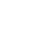loss函数随epoch的变化曲线

```callback
callback```

yinguobing/facial-landmark-detection-hrnet

A TensorFlow implementation of HRNet for facial landmark detection. – yinguobing/facial-landmark-detection-hrnetyinguobing GitHub### 构建定制化的callback类

TensorFlow使用callback类来实现训练过程中的人工干预。对于我们即将实现的callback对象，我们需要提供用于预测的样本图像以及日志路径。同时实例化一个日志写入器方便后续使用。

```class LogImages(keras.callbacks.Callback):
def __init__(self, logdir, sample_image):
super().__init__()
self.file_writer = tf.summary.create_file_writer(logdir)
self.sample_image = sample_image```

### 实现图像日志方法

`def on_epoch_end(self, epoch, logs={}):`

#### 读取图像并预处理

```# Read in the image file.
image = cv2.cvtColor(image, cv2.COLOR_BGR2RGB)
img = cv2.resize(image, (256, 256))
img = normalize(img)```

#### 馈入网络前向传播

`heatmaps = self.model.predict(tf.expand_dims(img, 0))`

#### 解析结果并记录

```marks, _ = parse_heatmaps(heatmaps, image.shape[:2])
for mark in marks:
cv2.circle(image, tuple(mark.astype(int)), 2, (0, 255, 0), -1)```

```with self.file_writer.as_default():
img_tensor = tf.expand_dims(image, 0)
tf.summary.image("test-sample", img_tensor, step=epoch)```